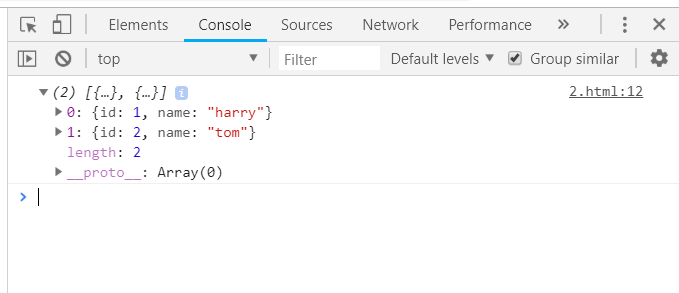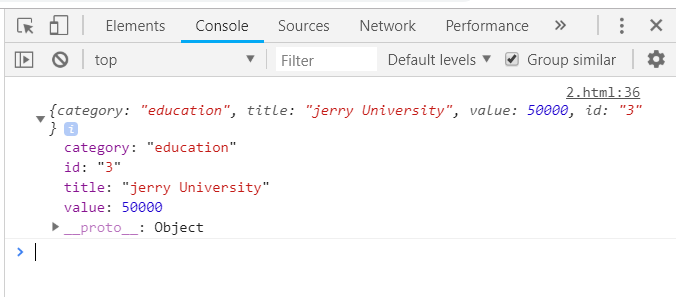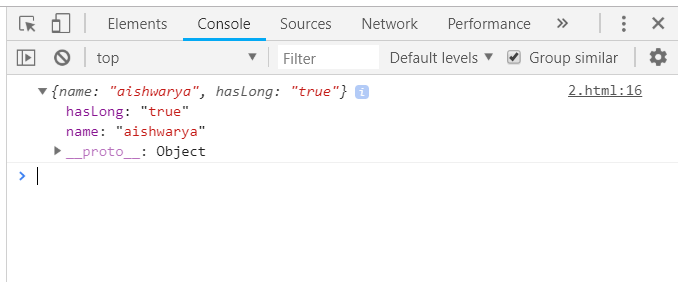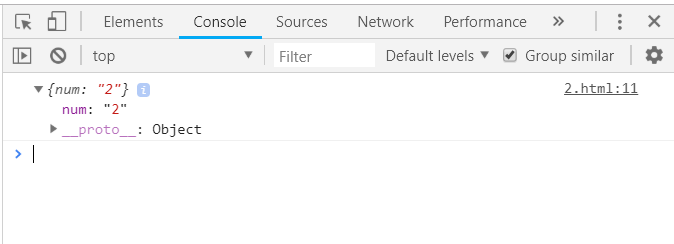Open in App
Not now

# Underscore.js _.whereWhere Function

• Last Updated : 24 Nov, 2021

The Underscore.js is a JavaScript library that provides a lot of useful functions that helps in the programming in a big way like the map, filter, invoke, etc even without using any built-in objects.

The _.findWhere() function is used to have a list of all the elements that matches the given property. The _.findWhere() function is used to search a content in the whole list of sections. The section which contains the content will be displayed.

Syntax:

```_.findWhere(list, properties)

```

Parameters: It takes two arguments:

• List: This parameter contains the list of items.
• Property: This parameter contains the test condition.

Return value: The details of the element selected from the list are returned. Only the first element matched will be given as output.

Difference between _.findWhere() and _.where() function: Both the functions takes an array name and the property to match but the _.where() function displays all the matches whereas, _.findWhere) function matches only the first match.

Searching a property in an array: The ._findWhere() function takes the array elements one by one and matches the given property is same or not. If the property matches it displays the rest of the details of that particular element. After the property is matched the first time the _.findWhere() function ends. It only displays the first match.

Example:

## HTML

 `<``html``>``    ``<``head``>``        ``<``title``>_.findWhere() function``        ``<``script` `type``=``"text/javascript"` `src``=``        ``"https://cdnjs.cloudflare.com/ajax/libs/underscore.js/1.9.1/underscore-min.js"` `>``        ````    ``       ``    ``<``body``>``        ``<``script` `type``=``"text/javascript"``>``            ``var users = [{id: 1, name: "harry"}, {id: 2, name: "jerry"}];``            ``_.findWhere(users, {id:2}).name = 'tom';``            ``console.log(users);``        ````    ``  ```

Output:Passing a list of elements with a number of different properties to _.findWhere() function: Firstly, declare the array with all the elements and their specific properties. Then pass the array name along with the property which needs to match to the _.findWhere() function. All the rest properties will be displayed as output of that specific element.

Example:

## HTML

 `<``html``>``    ``<``head``>``        ``<``title``>_.findWhere() function``        ``<``script` `type``=``"text/javascript"` `src``=``        ``"https://cdnjs.cloudflare.com/ajax/libs/underscore.js/1.9.1/underscore-min.js"` `>``        ````    ``       ``    ``<``body``>``        ``<``script` `type``=``"text/javascript"``>``            ``var goal  = [``                ``{``                    ``"category" : "other",``                    ``"title" : "harry University",``                    ``"value" : 50000,``                    ``"id":"1"``                ``},``                ``{``                    ``"category" : "traveling",``                    ``"title" : "tommy University",``                    ``"value" : 50000,``                    ``"id":"2"``                ``},``                ``{``                    ``"category" : "education",``                    ``"title" : "jerry University",``                    ``"value" : 50000,``                    ``"id":"3"``                ``},``                ``{    ``                    ``"category" : "business",``                    ``"title" : "Charlie University",``                    ``"value" : 50000,``                    ``"id":"4"``                ``}``            ``]``            ``console.log(_.findWhere(goal, {category: "education"}));``        ````    ``  ```

Output:Passing an array with ‘true/false’ as a property to the _.findWhere() function: First declare the array (here array is ‘people’). It is the property (here, ‘hasLong’) be defines as ‘true’ or ‘false’. Choose one condition to check like here ‘hasLongHairs’. Console.log display the final answer.

Example:

## HTML

 `<``html``>``    ``<``head``>``        ``<``title``>_.findWhere() function``        ``<``script` `type``=``"text/javascript"` `src``=``        ``"https://cdnjs.cloudflare.com/ajax/libs/underscore.js/1.9.1/underscore-min.js"` `>``        ````    ``       ``    ``<``body``>``        ``<``script` `type``=``"text/javascript"``>``            ``var people = [``                ``{"name": "sakshi", "hasLong": "false"},``                ``{"name": "aishwarya", "hasLong": "true"},``                ``{"name": "akansha", "hasLong": "true"},``                ``{"name": "preeti", "hasLong": "true"}``            ``]``         ``console.log(_.findWhere(people, {hasLong: "true"}));``        ````    `````

Output:Passing an array of numbers as property to _.findWhere() function together: It also follows the same procedure of firstly declaring the array with all the properties and the giving the array name and the property of matching to the _.findWhere() function.

Example:

## HTML

 `<``html``>``    ``<``head``>``        ``<``title``>_.findWhere() function``        ``<``script` `type``=``"text/javascript"` `src``=``        ``"https://cdnjs.cloudflare.com/ajax/libs/underscore.js/1.9.1/underscore-min.js"` `>``        ````    ``       ``    ``<``body``>``        ``<``script` `type``=``"text/javascript"``>``             ``var users = [{"num":"1"}, ``                          ``{"num":"2"}, ``                          ``{"num":"3"}, ``                          ``{"num":"4"}, ``                          ``{"num":"5"}];``        ``console.log(_.findWhere(users, {num:"2"}));``        ````    ````   ` ``

Output:My Personal Notes arrow_drop_up